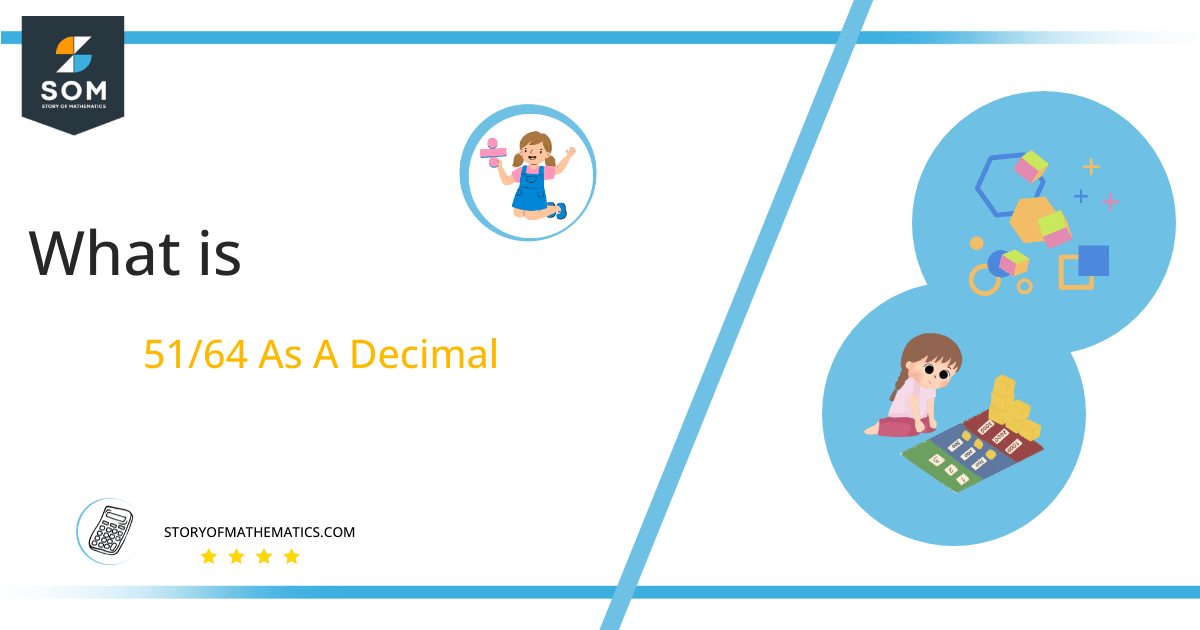# What Is 51/64 as a Decimal + Solution With Free Steps

The fraction 51/64 as a decimal is equal to 0.796.

Fractions are an expression that is a result of the division between two values. They are expressed as p/q, where q is the denominator and p is the numerator. In this expression ‘p‘ represents some number of parts out of ‘q‘ equal number of parts.

Here, we are more interested in the division types that result in a Decimal value, as this can be expressed as a Fraction. We see fractions as a way of showing two numbers having the operation of Division between them that result in a value that lies between two Integers.Now, we introduce the method used to solve said fraction to decimal conversion, called Long Division, which we will discuss in detail moving forward. So, let’s go through the Solution of fraction 51/64.

## Solution

First, we convert the fraction components, i.e., the numerator and the denominator, and transform them into the division constituents, i.e., the Dividend and the Divisor, respectively.

This can be done as follows:

Dividend = 51

Divisor = 64

Now, we introduce the most important quantity in our division process: the Quotient. The value represents the Solution to our division and can be expressed as having the following relationship with the Division constituents:

Quotient = Dividend $\div$ Divisor = 51 $\div$ 64

This is when we go through the Long Division solution to our problem. The solution can be seen in figure 1.Figure 1

## 51/64 Long Division Method

We start solving a problem using the Long Division Method by first taking apart the division’s components and comparing them. As we have 51 and 64, we can see how 51 is Smaller than 64, and to solve this division, we require that 51 be Bigger than 64.

This is done by multiplying the dividend by 10 and checking whether it is bigger than the divisor or not. If so, we calculate the Multiple of the divisor closest to the dividend and subtract it from the Dividend. This produces the Remainder, which we then use as the dividend later.

Now, we begin solving for our dividend 51, which after getting multiplied by 10 becomes 510.

We take this 510 and divide it by 64; this can be done as follows:

510 $\div$ 64 $\approx$ 7

Where:

64 x 7 = 448

This will lead to the generation of a Remainder equal to 510 – 448 = 62. Now this means we have to repeat the process by Converting the 62 into 620 and solving for that:

620 $\div$ 64 $\approx$ 9

Where:

64 x 9 = 576

This, therefore, produces another Remainder equal to 620 – 576 = 44. Now we must solve this problem for the Third Decimal Place for accuracy, so we repeat the process with dividend 440.

440 $\div$ 64 $\approx$ 6

Where:

64 x 6 = 384

Finally, we have a Quotient generated after combining the three pieces of it as 0.796, with a Remainder equal to 56.Images/mathematical drawings are created with GeoGebra.